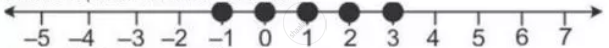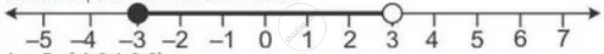Share

# Given: a = {X: -8 < 5x + 2 ≤ 17, X ∈ I}, B = {X: -2 ≤ 7 + 3x < 17, X ∈ R} Where R = {Real Numbers} and I = {Integers}. Represent a and B on Two Different Number Lines. Write Down the Elements of a ∩ B. - Mathematics

Course

#### Question

Given: A = {x: -8 < 5x + 2 ≤ 17, x ∈ I}, B = {x: -2 ≤ 7 + 3x < 17, x ∈ R}
Where R = {real numbers} and I = {integers}. Represent A and B on two different number lines. Write down the elements of A ∩ B.

#### Solution

A = {x :-8 < 5x + 2  <= 17,  x ∈ I}

= {x :-10 < 5x <= 15, x  ∈ I}

= {x : -2 < x <= 3, x ∈ I}

It can be represented on number line asB = {x : -2 <= 7 + 3x < 17, x ∈ R}

= {x : 9 <= 3x < 10, x ∈ R}

={x : -3 <= x < 3.33, x ∈ R}

it can be represeneted on number line asA ∩ B = {-1, 0, 1, 2, 3}

Is there an error in this question or solution?

#### APPEARS IN

Selina Solution for Concise Mathematics for Class 10 ICSE (2020 (Latest))
Chapter 4: Linear Inequations (In one variable)
Exercise 4(B) | Q: 21 | Page no. 50
Solution Given: a = {X: -8 < 5x + 2 ≤ 17, X ∈ I}, B = {X: -2 ≤ 7 + 3x < 17, X ∈ R} Where R = {Real Numbers} and I = {Integers}. Represent a and B on Two Different Number Lines. Write Down the Elements of a ∩ B. Concept: Representation of Solution on the Number Line.
S